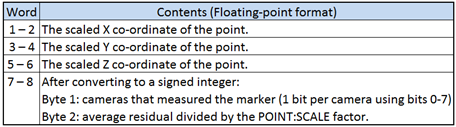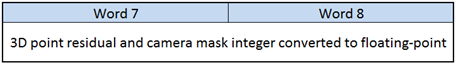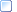﻿ 3D Data - Floating-point Format
 The 3D Point Data Section > Description

### 3D Data - Floating-point Format

If the POINT:SCALE parameter is negative then the 3D data section will contain scaled floating-point format data for each stored trajectory.  This format provides increased precision and, since the data is stored as scaled values, the POINT:SCALE parameter is not used to apply a scaling factor to the data.  It is however used to calculate the point residual value and may be applied to the data if the file is converted to an integer format so it is important to calculate a valid POINT:SCALE factor when 3D points are stored as floating-point values.  Since the floating-point format require eight 16-bit words to store a single 3D point, it will result in C3D files that are double the size of integer format C3D files.

Please note that a valid scaling factor is always required as it is used in the calculation of the 3D point residual value.  It should be calculated, based on the maximum coordinate value and not just set to -1.Figure 24 - 3D point data storage using floating-point format.

The first three floating-point words record the scaled X, Y, and Z coordinate values of the 3D data point.  Word four is a floating-point value that must be converted to a signed integer and then interpreted as two bytes.  The first byte stores which observers (normally cameras) provided information used in calculating the 3D point, while the second byte contains the residual of the 3D point measurement.Figure 25- Residual and mask storage – Floating-point format.

The camera mask is optional but the 3D point residual is a measurement that, if calculated and stored correctly, provides important information about the relative accuracy of each individual 3D measurement recorded in the C3D file.  A valid residual indicates that the 3D coordinate is a measurement; a negative residual value indicates that the 3D coordinates are invalid, while a residual set to zero indicates that the 3D coordinate has been processed and is not the original measured value.

More:Notes for programmers - Floating-point 3D Data